Maths-
General
Easy

###Hint:

The definition of a quadratic as a second-degree polynomial equation demands that at least one squared term must be included. It also goes by the name quadratic equations. Here we have to find the cartesian equation of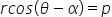.

#### A quadratic equation, or sometimes just quadratics, is a polynomial equation with a maximum degree of two. It takes the following form:ax² + bx + c = 0where a, b, and c are constant terms and x is the unknown variable.Now we have given the equation as.Now we know that: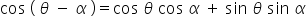.So applying this, we get: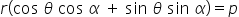Now lets substitute x=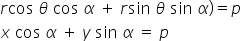Here we used the concept of quadratic equations and solved the problem. We also understood the concept of trigonometric ratios and used the formula to find the equation. So the equation is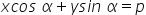.

## Book A Free Demo+91